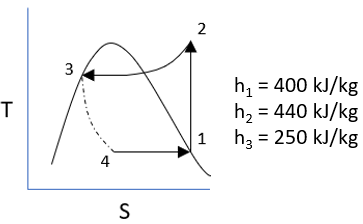## Refrigeration Cycle

The below figure describes a refrigeration cycle using R-12a and its associated enthalpies. What is the coefficient of performance (COP)?Hint
$$COP=\frac{h_1-h_4}{h_2-h_1}$$$Hint 2 Since this is a refrigeration cycle, $$h_4=h_3$$ . $$COP=\frac{h_1-h_4}{h_2-h_1}$$$
Note that $$h_4=h_3$$ due to the fact this is a refrigeration cycle. Thus,
$$COP=\frac{(400-250)}{(440-400)}\cdot \frac{kJ/kg}{kJ/kg}=\frac{150}{40}=3.75$$\$
3.75